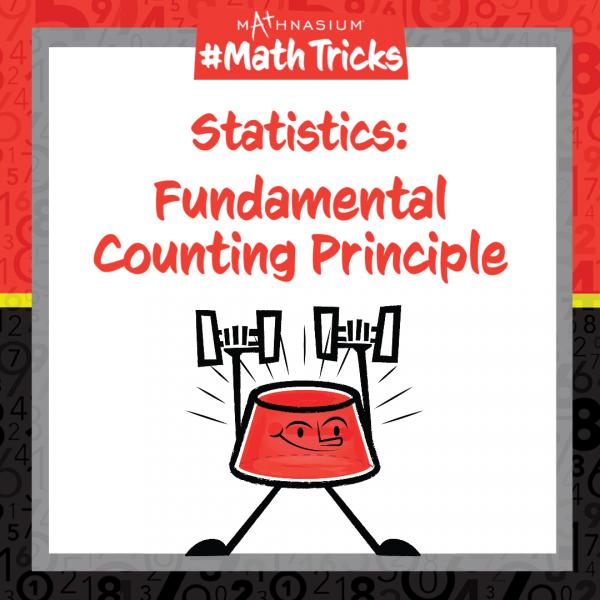# Mathnasium #MathTricks: Statistics (Counting Part 1)

By Mathnasium | Added Apr 20, 2022Welcome to Mathnasium’s Math Tricks series. Whether it is in statistics or the real world, we are occasionally required to count large amounts. It can be a long and tedious process to list the total number of combinations for given events. So today we are showing you how to use the Fundamental Counting Principle to count the number of outcomes; the trick is to multiply the number of possibilities for each event together.

Use the Fundamental Counting Principle to find the number of combinations in the example below.

# Example: How many 3-digit numbers satisfy all of the following conditions?

The first digit is greater than 6.

The second digit is an odd number.

The number is divisible by 2.

Step 1: Identify the possibilities for each condition.

The first digit can be: 7, 8, 9.

The second digit can be: 1, 3, 5, 7, 9.

The 3-digit number must end in an even number to be divisible by two: 0, 2, 4, 6, 8.

Step 2: Identify the number of possibilities for each condition.

The first digit has 3 possibilities.

The second digit has 5 possibilities.

The third digit has 5 possibilities.

Step 3: Multiply the number of possibilities for each condition.

3 x 5 x 5 = 75